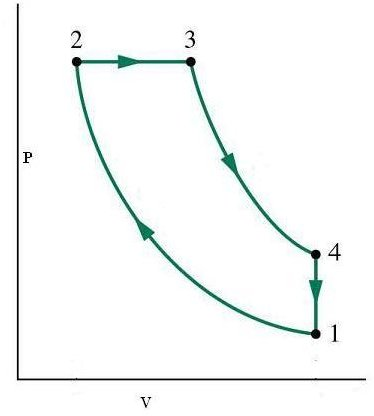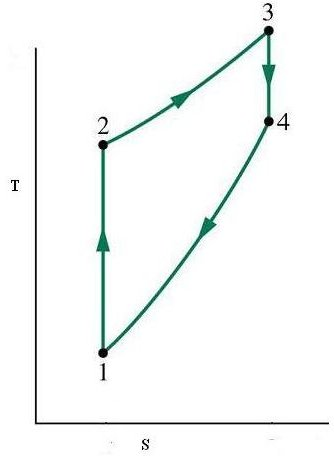# Theoretical Cycles in Marine Diesel Engines – The Diesel Cycle

## Introduction

We studied about the Otto cycle in the previous article which you can read by clicking here, and in this article we will move further by studying the diesel cycle upon which Rudolf Diesel based his initial diesel engine. As before, just remember that these are ideal cycles not fully followed by real engines and use air as the standard working substance.

## The Diesel Cycle

The four processes of this cycle as explained as follows with the accompanying P-V and T-S diagrams for clarity.

1-2 represents the isentropic compression of air leading to rise in temperature and pressure and significant reduction in volume. This reduction in volume or the ratio of reduction is known as compression ratio. This corresponds to the suction stroke of the real engine

2-3 is the addition of heat at a constant pressure corresponding to ignition in the real engine, leading to rise in temperature and entropy and in volume as well. Just remember from your previous study of the Otto cycle that this head addition was at a constant volume over there, hence the difference

3-4 is the isentropic expansion of air corresponding to power stroke and leads to reduction in temperature, pressure and volume.

4-1 is the rejection of heat at a constant volume (same as the Otto) and at the end of this process the working substances reaches the same point from where it started thus completing one full cycle of four strokes.

The thermal efficiency is calculated like before by dividing the amount of heat converted into work by the total amount of heat supplied and it gives

Thermal Efficiency = 1 – 1/k (T4 – T1/T3 – T2)

It is also calculated using the gas laws and can be written in the following format as well

Thermal Efficiency = 1 – (1/r)^(k-1) * {((rc^k) – 1)/k(rc – 1)}

Where r is the compression ratio between points 2 and 1, and rc is the compression ratio between the points 3 and 2, k being dependent on the working substance and is a constant

Hence you can see that the thermal efficiency of the diesel cycle is different from the thermal efficiency of the Otto cycle by the figure in the curly brackets. That figure is always greater than unity hence the efficiency of the diesel engine will be lesser than that of the Otto cycle. The only way to overcome this deficiency is to use higher compression ratios in case of real engines to offset the effect of lower efficiency. Just to give an example the thermal efficiency of a diesel cycle engine having a compression ratio of 14 would be greater than an Otto cycle engine having compression ratio of 7 due to this offset effect.

## P-V and T-S Diagrams